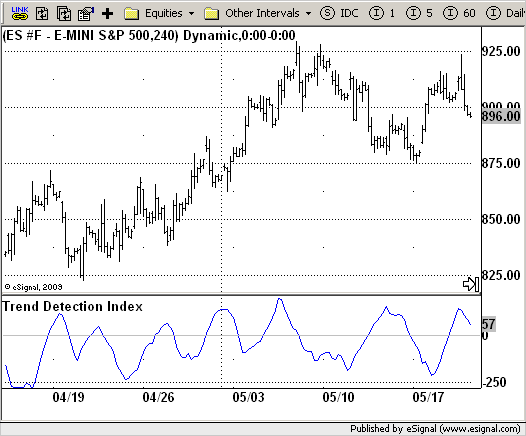# Trend Direction Index (TDI)

ICE Data Services -

 TDI.efs EFSLibrary - Discussion Board

File Name: TDI.efs

Description:
TDI (Trend Direction Index)

Formula Parameters:
Period : 12

Notes:
The Trend Detection Index (TDI) was introduced by M. H. Pee. TDI is used
to detect when a trend has begun and when it has come to an end. The TDI
can be used as a stand-alone indicator or combined with others; it will
perform well in detecting the beginning of trends. TDI should be used in
conjunction with protective stops as well as trailing stops. These stops
are required to protect against large losses when the indicator generates
a losing trade. The TDI can trade a diverse portfolio of markets profitably
over many years, using the same parameters throughout.
To calculate the 20-day trend detection index, first find the value of the
momentum indicator. After the market closes, calculate today's 20-day momentum
by subtracting the close 20 days ago from that of today. Next, find the 20-day
absolute momentum, which is defined as the absolute value of today's 20-day momentum.
More details can be found in the formula section above.
The trend detection index will signal a trend if it shows a positive value and a
consolidation if it shows a negative value. As a trend-follower, the position should
be entered in the direction of the trend when the TDI is positive. To determine the
current direction of the trend, the direction indicator can be used, which is defined
as the sum of the 20-day momentum of the last 20 days. An uptrend is signaled by a positive
direction indicator value, whereas a downtrend is signaled by a negative value. Basically,
it comes down to this: Enter long tomorrow at the open if both the TDI and direction indicator
are positive after today's close or enter short at the open if the TDI is positive and the
direction indicator is negative.`/*********************************Provided By: eSignal (Copyright c eSignal), a division of Interactive Data Corporation. 2009. All rights reserved. This sample eSignal Formula Script (EFS) is for educational purposes only and may be modified and saved under a new file name. eSignal is not responsible for the functionality once modified. eSignal reserves the right to modify and overwrite this EFS file with each new release.Description: TDI (Trend Direction Index) Version: 1.0 05/19/2009 Formula Parameters: Default: Period 12 Notes: The Trend Detection Index (TDI) was introduced by M. H. Pee. TDI is used to detect when a trend has begun and when it has come to an end. The TDI can be used as a stand-alone indicator or combined with others; it will perform well in detecting the beginning of trends. TDI should be used in conjunction with protective stops as well as trailing stops. These stops are required to protect against large losses when the indicator generates a losing trade. The TDI can trade a diverse portfolio of markets profitably over many years, using the same parameters throughout. To calculate the 20-day trend detection index, first find the value of the momentum indicator. After the market closes, calculate today's 20-day momentum by subtracting the close 20 days ago from that of today. Next, find the 20-day absolute momentum, which is defined as the absolute value of today's 20-day momentum. More details can be found in the formula section above. The trend detection index will signal a trend if it shows a positive value and a consolidation if it shows a negative value. As a trend-follower, the position should be entered in the direction of the trend when the TDI is positive. To determine the current direction of the trend, the direction indicator can be used, which is defined as the sum of the 20-day momentum of the last 20 days. An uptrend is signaled by a positive direction indicator value, whereas a downtrend is signaled by a negative value. Basically, it comes down to this: Enter long tomorrow at the open if both the TDI and direction indicator are positive after today's close or enter short at the open if the TDI is positive and the direction indicator is negative. **********************************/var fpArray = new Array();var bInit = false;function preMain(){ setStudyTitle("Trend Detection Index"); setCursorLabelName("TDI",0); setDefaultBarFgColor(Color.blue,0); addBand(0, PS_SOLID, 1, Color.lightgrey); var x = 0; fpArray[x] = new FunctionParameter("Period", FunctionParameter.NUMBER); with(fpArray[x++]) { setLowerLimit(1); setDefault(12); } }var xTDI = null;function main(Period) {var nBarState = getBarState();var nTDI = 0; if (nBarState == BARSTATE_ALLBARS) { if(Period == null) Period = 12; } if (bInit == false) { xTDI = efsInternal("Calc_TDI", Period); bInit = true; } nTDI = xTDI.getValue(0); if (nTDI == null) return; return nTDI;}var bSecondInit = false;var xmom = null;function Calc_TDI(Period) {var nRes = 0;var MomSum = 0;var MomSumAbs = 0;var MomAbsSum = 0;var MomAbsSum2 = 0; if (getCurrentBarCount() <= Period * 2) return; if (bSecondInit == false) { xmom = mom(Period); bSecondInit = true; } for(i = Period * 2; i--; i >= 0) { nRes = xmom.getValue(-i); if (i < Period) { MomSum += nRes; MomAbsSum += Math.abs(nRes); } MomAbsSum2 += Math.abs(nRes); } MomSumAbs = Math.abs(MomSum); nRes = MomSumAbs - (MomAbsSum2 - MomAbsSum); return nRes;}`